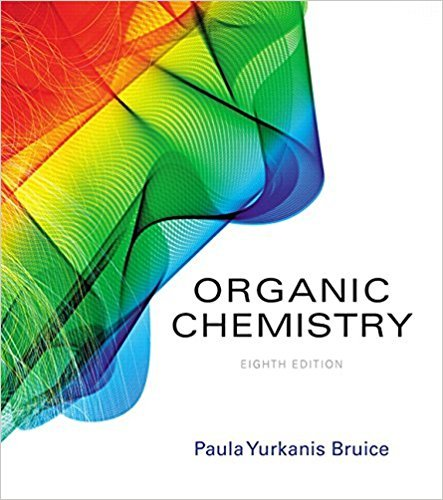×
×

# Solutions for Chapter 1.5: Atomic Orbitals## Full solutions for Organic Chemistry | 8th Edition

ISBN: 9780134042282Solutions for Chapter 1.5: Atomic Orbitals

Solutions for Chapter 1.5
4 5 0 333 Reviews
31
4
##### ISBN: 9780134042282

Organic Chemistry was written by and is associated to the ISBN: 9780134042282. Since 4 problems in chapter 1.5: Atomic Orbitals have been answered, more than 28649 students have viewed full step-by-step solutions from this chapter. Chapter 1.5: Atomic Orbitals includes 4 full step-by-step solutions. This expansive textbook survival guide covers the following chapters and their solutions. This textbook survival guide was created for the textbook: Organic Chemistry, edition: 8.

Key Chemistry Terms and definitions covered in this textbook
• alcohol

An organic compound obtained by substituting a hydroxyl group 1¬OH2 for a hydrogen on a hydrocarbon. (Sections 2.9 and 24.4)

• aldose

A carbohydrate that contains an aldehyde group.

• Allene

The compound CH2"C"CH2. Any compound that contains adjacent carbon-carbon double bonds; that is, any molecule that contains a C"C"C functional group.

• allylic

The positions that are adjacent to the vinylic positions of a carboncarbon double bond.

• chiral

An object that is not superimposable on its mirror image.

• chiral

A term describing a molecule or an ion that cannot be superimposed on its mirror image. (Sections 23.4 and 24.5)

• complex lipid

A lipid that readily undergoes hydrolysis in aqueous acid or base to produce smaller fragments.

• condensation polymerization

Polymerization in which molecules are joined together through condensation reactions. (Section 12.8)

• Dalton’s law of partial pressures

A law stating that the total pressure of a mixture of gases is the sum of the pressures that each gas would exert if it were present alone. (Section 10.6)

• Dehydrohalogenation

Removal of !H and !X from adjacent carbons; a type of b-elimination

• deoxyribonucleic acids (DNA).

A type of nucleic acid. (25.4)

• double helix

The structure for DNA that involves the winding of two DNA polynucleotide chains together in a helical arrangement. The two strands of the double helix are complementary in that the organic bases on the two strands are paired for optimal hydrogen bond interaction. (Section 24.10)

• Enthalpy change, DH

The difference in total bond strengths and solvation between various points under comparison on a reaction coordinate diagram

• functional group

A characteristic group of atoms/bonds that possess a predictable chemical behavior.

• group

Elements that are in the same column of the periodic table; elements within the same group or family exhibit similarities in their chemical behavior. (Section 2.5)

• intermediate

A structure corresponding to a local minimum (valley) in an energy diagram.

• nonelectrolyte

A substance that does not ionize in water and consequently gives a nonconducting solution. (Section 4.1)

• parts per billion (ppb)

The concentration of a solution in grams of solute per 109 (billion) grams of solution; equals micrograms of solute per liter of solution for aqueous solutions. (Section 13.4)

• scientific law

A concise verbal statement or a mathematical equation that summarizes a wide range of observations and experiences. (Section 1.3)

• Thermodynamic control

Experimental conditions that permit the establishment of equilibrium between two or more products of a reaction. The composition of the product mixture is determined by the relative stabilities of the products.

×